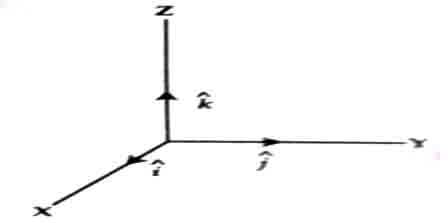# Vector Symbols: Major Types

A vector quantity is expressed in two ways by sign; e.g. by letter or by straight line. By letter a vector quantity can be divided in four ways; e.g.:

(a) Vector form of a quantity is expressed by putting an arrow over a letter and its magnitude is expressed by placing two vertical lines across the letter. Normally, magnitude of the quantity is also expressed only by the letter

so, vector form of word A is Â and its magnitude is ǀÂǀ or simply A.

(b) Vector quantity is expressed by putting a line above a letter and its magnitude is expressed by two vertical lines across the letter.

so, vector form of A is Ᾱ and its magnitude is ǀᾹǀ.

(c) Vector quantity is represented by putting a line underneath the letter and its magnitude is expressed by two vertical lines across that letter. For example, vector representation of A is Ḁ and its magnitude is ǀḀǀ

(d) A vector quantity is represented by a bold letter. For example vector form of A is A and its magnitude is A.In three dimensional coordinate system, rectangular units vectors are shown by symbols î, ĵ and ƙ along the axes, X, Y and Z (fig).